Chemical Equilibrium Short Notes for Class 11, JEE & NEET
eSaral provides chemistry short notes for JEE and NEET to help students in revising topics quickly. These notes are completely based on latest syllabus and it includes all the tips and tricks that will help you in learning chemistry better and score well.The Notes will help you to understand the important topics and remember the key points for exam point of view.You can also access detailed Notes of chemistry here.Download or View Detailed Notes for Chemistry Class 11thDownload or View Detailed Notes for Chemistry Class 12thEquivalent Concept Short Notes for Class 11, JEE & NEET
eSaral provides chemistry short notes for JEE and NEET to help students in revising topics quickly. These notes are completely based on latest syllabus and it includes all the tips and tricks that will help you in learning chemistry better and score well.The Notes will help you to understand the important topics and remember the key points for exam point of view.You can also access detailed Notes of chemistry here.Download or View Detailed Notes for Chemistry Class 11thDownload or View Detailed Notes for Chemistry Class 12thIonic Equilibrium Short Notes for Class 11, JEE & NEET
eSaral provides chemistry short notes for JEE and NEET to help students in revising topics quickly. These notes are completely based on latest syllabus and it includes all the tips and tricks that will help you in learning chemistry better and score well.The Notes will help you to understand the important topics and remember the key points for exam point of view.You can also access detailed Notes of chemistry here.Download or View Detailed Notes for Chemistry Class 11thDownload or View Detailed Notes for Chemistry Class 12th Ionic Equilibrium TheoryFundamentals of Acids, Bases & Ionic Equilibrium Acids & BasesWhen dissolved in water, acids release $\mathrm{H}^{+}$ ions, base release $\mathrm{OH}^{-}$ ions. Arrhenius TheoryWhen dissolved in water, the substances which release(i) $\mathrm{H}^{+}$ ions are called acids (ii) $\mathrm{OH}^{-}$ ions are called bases Bronsted & Lowry ConceptAcids are proton donors, bases are proton acceptorsNote that as per this definition, water is not necessarily the solvent. When a substance is dissolved in water, it is said to react with water e.g.$\mathrm{HCl}+\mathrm{H}_{2} \mathrm{O} \rightarrow \mathrm{H}_{3} \mathrm{O}^{+}+\mathrm{Cl}^{-}$ ; HCl donates H+ to water, hence acid.$\mathrm{NH}_{3}+\mathrm{H}_{2} \mathrm{O} \quad \rightarrow \mathrm{NH}_{4}^{+}+\mathrm{OH}^{-}$ ; NH3 takes H+ from water, hence base.For the backward reaction, $N H_{4}^{+}$ donate $H^{+}$ , hence it is an acid; $O H^{-}$ accepts H+, hence it is base. $N H_{3}$ (base) & $N H_{4}^{+}$ (acid) from conjugate acid base pair.Conjugate acid and basesTo get conjugate acid of a given species add $\mathrm{H}^{+}$ to it. e.g. conjugate acid of N2H4 is N2H5+.To get conjugate base of any species subtract H+ from it. e.g. Conjugate base of $\mathrm{NH}_{3}$ is $\mathrm{NH}_{2}^{-}$. Note: Although $C I^{-}$ is conjugate base of HCl, it is not a base as an independent species. In fact,anions of all strong acid like $C I, N O_{3}^{-}, C l O_{4}^{-}$ etc. are neutral anions. Same is true for cations of strong bases like $K^{+}, N a^{+}, B a^{++}$ etc. When they are dissolved in water, they do not react with water (i.e. they do not undergo hydrolysis) and these ions do not cause any change in pH of water (others like $\left.C N^{-} d o\right)$.Some examples of :Basic Anions : $\mathrm{CH}_{3} \mathrm{COO}^{-}, \mathrm{OH}^{-}, \mathrm{CN}^{-}$ (Conjugate bases of weak acids) Acid Anions: $H S O_{3}^{-}, H S^{-}$ etc. Note that these ions are amphoteric, i.e. they can behave both as an acid and as a base. e.g. for $\mathrm{H}_{2} \mathrm{PO}_{4}^{-}:$Lewis Concept : Acids are substances which accept a pair of electrons to form a coordinate bond and bases are the substances which donate a pair of electrons to form a coordinate bond.Important : $\mathrm{Ca}+\mathrm{S} \rightarrow \mathrm{Ca}^{2+}+\mathrm{S}^{2-}$ is not a Lewis acidbase reaction since dative bond is not formed.Lewis Acids : As per Lewis concept, following species can acts as Lewis Acids :(i) Molecules in which central atom has incomplete octet. \text { (e.g. }\left.\mathrm{BF}_{3}, \mathrm{AlCl}_{3} \text { etc. }\right)(ii) Molecules which have a central atom with empty d orbitals \text { (e.g. }\left.\operatorname{six}_{4}, \mathrm{GeX}_{4}, \mathrm{PX}_{3}, \mathrm{TiCl}_{4} \text { etc. }\right)(iii) Simple Cations: Though all cations can be expected to be Lewis acids, \mathrm{Na}^{+}, \mathrm{Ca}^{++}, \mathrm{K}^{+} \text {etc. } show no tendency to accept electrons. However \mathrm{H}^{+}, \mathrm{Ag}^{+} etc. act as Lewis acids.(iv) Molecules having multiple bond between atoms of dissimilar electronegativity.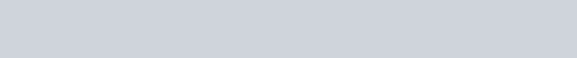Lewis bases are typically :(i) Neutral species having at least one lone pair of electrons.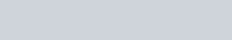Autoprotolysis of water (or any solvent)\text { Autoprotolysis (or self-ionization) constant }\left(\mathrm{K}_{\mathrm{w}}\right)=\left[\mathrm{H}_{3} \mathrm{O}^{+}\right]\left[\mathrm{OH}^{-}\right]\text { Hence, } \mathrm{pH}+\mathrm{pOH}=\mathrm{pK}_{\mathrm{w}} \text { at all temperatures }Condition of neutrality \left[\mathrm{H}_{3} \mathrm{O}^{+}\right]=\left[\mathrm{OH}^{-}\right] \text {(for water as solvent) }\text { At } 25^{\circ} \mathrm{C}, \mathrm{K}_{\mathrm{W}}=10^{-14} \cdot \mathrm{K}_{\mathrm{W}}increases with increase in temperature. Accordingly, the neutralpoint of water \left(\mathrm{pH}=7 \text { at } 25^{\circ} \mathrm{C}\right) also shifts to a value lower than 7 with increase in temperature. Important: \mathrm{K}_{\mathrm{W}}=10^{-14} s a value at \text { (i) } 25^{\circ} \mathrm{C} \text { (ii) }for water only. If the temperature changes or if some other solvent is used, autoprotolysis constant will not be same.Ionisation Constant* \quad \text { For dissociation of weak acids (eg. HCN), HCN + H_{ } 2 } \mathrm{O} \text { 1 } \mathrm{H}_{3} \mathrm{O}^{+}+\mathrm{CN}^{-} \text {the equilibrium }constant expression is written as Ka =\frac{\left[\mathrm{H}_{3} \mathrm{O}^{+}\right]\left[\mathrm{CN}^{-}\right]}{[\mathrm{HCN}]}* For the Polyprotic acids \left(\mathrm{e} \cdot \mathrm{g} \cdot \mathrm{H}_{3} \mathrm{PO}_{4}\right) sucessive ionisation constants are denoted \text { by } \mathrm{K}_{1}, \mathrm{K}_{2}, \mathrm{K}_{3} \text { etc. } For \mathrm{H}_{3} \mathrm{PO}_{4},\text { Similarly, } \mathrm{K}_{\mathrm{b}} \text { denotes basic dissociation constant for a base. }\text { Also, } \mathrm{pK}_{\mathrm{a}}=-\log _{10} \mathrm{K}_{\mathrm{a}}, \mathrm{pK}_{\mathrm{b}}=-\log _{10} \mathrm{K}_{\mathrm{b}}\text { Some Important Results: }\left[\mathrm{H}^{+}\right] \text {concentration of }Case (i) A weak acid in waterSimilarly for a weak base, substitute \left[\mathrm{OH}^{-}\right] \text {and } \mathrm{K}_{\mathrm{b}} instead of \left[\mathrm{H}^{+}\right] \text {and } \mathrm{K}_{\mathrm{a}}respectively in these expressions. Case (ii) (a) A weak acid and a strong acid \left[\mathrm{H}^{+}\right] is entirely due to dissociation of strong acid(b) A weak base and a strong base \left[\mathrm{H}^{+}\right] is entirely due to dissociation of strong base Neglect the contribution of weak acid/base usually.Condition for neglecting : \text { If } \mathrm{c}_{0} concentration of strong acid, c_{1} = concentration of weak acid then neglect the contribution of weak acid if \mathrm{K}_{\mathrm{a}} \leq 0.01 \mathrm{c}_{0}^{2 /} \mathrm{c}_{1} Case (iii) Two (or more) weak acidsProceed by the general method of applying two conditions(i) of electroneutrality (ii) of equilibria. The accurate treatement yields a cubic equation. Assuming that acids dissociate to\text { a negligible extent }\left[\text { i.e. } c_{0}-x \approx c_{0}\right] \quad\left[\mathrm{H}^{+}\right]=\left(\mathrm{K}_{1} \mathrm{c}_{1}+\mathrm{K}_{2} \mathrm{c}_{2}+\ldots+\mathrm{K}_{\mathrm{w}}\right)^{1 / 2}Case (iv) When dissociation of water becomes significant:\text { Dissociation of water contributes significantly to }\left[\mathrm{H}^{+}\right] \text {or }\left[\mathrm{OH}^{-}\right] \text {only when for }(i) strong acids (or bases) : 10^{-8} \mathrm{M}<\mathrm{c}_{0}<10^{-6} \mathrm{M}. Neglecting ionisation of water at10^{-6} \mathrm{M} \text { causes } 1 \% \text { error (approvable). Below } 10^{-8} \mathrm{M}, Neglecting ionisation of water at 10^{-6} \mathrm{M} \text { causes } 1 \% \text { error (approvable). } Below 10^{-8} \mathrm{M}, contribution of acid (or base) can be neglected and pH can be taken to be practically 7.Weak acids (or bases) : \text { When } \mathrm{K}_{\mathrm{a}} \mathrm{c}_{0}<10^{-12}then consider dissociation of water as well. HYDROLYSIS* Salts of strong acids and strong bases do not undergo hydrolysis.* Salts of a strong acids and weak bases give an acidic solution. e.g. \mathrm{NH}_{4} \mathrm{Cl} when dissolved, it dissociates to give \mathrm{NH}_{4}^{+} ions and \mathrm{NH}_{4}^{+}+\mathrm{H}_{2} \mathrm{O} \quad 1 \quad \mathrm{NH}_{3}+\mathrm{H}_{3} \mathrm{O}^{+}\mathrm{K}_{\mathrm{h}}=\left[\mathrm{NH}_{3}\right]\left[\mathrm{H}_{3} \mathrm{O}^{+}\right] /\left[\mathrm{NH}_{4}^{+}\right]=\mathrm{K}_{\mathrm{W}} / \mathrm{K}_{\mathrm{b}} of conjugate base of \mathrm{NH}_{4}^{+}Important! In general : \mathrm{K}_{\mathrm{a}}(\text { of an acid }) \mathrm{xK}_{\mathrm{b}} \text { (of its conjugate base) }=\mathrm{K}_{\mathrm{w}}If the degree of hydrolysis(h) is small (<<1), \quad \mathrm{h}=\sqrt{\mathrm{K}_{\mathrm{h}} \mathrm{c}_{0}}Otherwise h = \frac{-\mathrm{K}_{\mathrm{h}}+\sqrt{\mathrm{K}_{\mathrm{h}}^{2}+4 \mathrm{K}_{\mathrm{h}} \mathrm{c}_{0}}}{2 \mathrm{c}_{0}}, \quad\left[\mathrm{H}^{+}\right]=\mathrm{c}_{0} \mathrm{h}* Salts of strong base and weak acid give a basic solution (\mathrm{pH}>7) when dissolved in water, e.g. NaCN,\mathrm{CN}^{-}+\mathrm{H}_{2} \mathrm{O} \quad 1 \quad \mathrm{HCN}+\mathrm{OH}^{-} \quad\left[\mathrm{OH}^{-}\right]=\mathrm{c}_{0} \mathrm{h}, \mathrm{h}=\sqrt{\mathrm{K}_{\mathrm{h}} \mathrm{c}_{0}}* Salts of weak base and weak acidAssuming degree of hydrolysis to be same for the both the ions,\mathrm{K}_{\mathrm{h}}=\mathrm{K}_{\mathrm{w}} /\left(\mathrm{K}_{\mathrm{a}} \cdot \mathrm{K}_{\mathrm{b}}\right),\left[\mathrm{H}^{+}\right]=\left[\mathrm{K}_{\mathrm{a}} \mathrm{K}_{\mathrm{w}} / \mathrm{K}_{\mathrm{b}}\right]^{1 / 2}Note: Exact treatment of this case is difficult to solve. So use this assumption in general cases.Also, degree of anion or cation will be much higher in the case of a salt of weak acid and weak base. This is because each of them gets hydrolysed, producing \mathrm{H}^{+} \text {and } \mathrm{OH}^{-} ions. These ions combine to form water and the hydrolysis equilibrium is shifted in the forward direaction. Buffer Solutions are the solutions whose pH does not change significantly on adding a small quantity of strong base or on little dilution.These are typically made by mixing a weak acid (or base) with its conjugate base (or acid). e.g. \mathrm{CH}_{3} \mathrm{COOH} with \mathrm{CH}_{3} \mathrm{COONa}, \mathrm{NH}_{3}(\mathrm{aq}) \text { with } \mathrm{NH}_{4} \mathrm{Cl} \text { etc. }\text { If }\left.\mathrm{K}_{\mathrm{a}} \text { for acid (or } \mathrm{K}_{\mathrm{b}} \text { for base }\right) (or Kb for base) is not too high, we may write :Henderson’s Equation\mathrm{pH}=\mathrm{pK}_{\mathrm{a}}+\log \{[\mathrm{salt}] /[\mathrm{acid}]\} for weak acid with its conjugate base.\text { or } \mathrm{pOH}=\mathrm{pK}_{\mathrm{b}}+\log \{[\mathrm{salt}] /[\text { base }]\} for weak base with its conjugate acid.Important : For good buffer capacity, [salt] : [acid ratios should be as close to one as possible. In such a case, \mathrm{pH}=\mathrm{pK}_{\mathrm{a}} (This also is the case at midpoint of titration)Buffer capacity = (no. of moles of acid (or base) added to 1L) / (change in pH)Indicators. Indicator is a substance which indicates the point of equivalence in a titration by undergoing a change in its colour. They are weak acids or weak bases.Theory of Indicators. The ionized and unionized forms of indicators have different colours. If 90 % or more of a particular form (ionised or unionised) is present, then its colour can be distinclty seen.In general, for an indicator which is weak acid, HIn l H+ + In–, the ratio of ionized to unionized form can be determined fromThis roughly gives the range of indicators. Ranges for some popular indicators areTable 1 : IndicatorsEquivalence point. The point at which exactly equivalent amounts of acid and base have been mixed. Acid Base Titration. For choosing a suitable indicator titration curves are of great help. In a titration curve, change in pH is plotted against the volume of alkali to a given acid. Four cases arise.(a) Strong acid vs strong base. The curve is almost vertical over the pH range 3.5-10. This abrupt change corresponds to equivalence point. Any indicator suitable.(b) Weak acid vs strong base. Final solution is basic 9 at equivalence point. Vertical region (not so sharp) lies in pH range 6.5-10. So, phenolphathlene is suitable.(c) Strong acid vs weak base. Final solution acidic. Vertical point in pH range 3.8-7.2. Methyl red or methyl orange suitable. (d) Weak acid vs weak base. No sharp change in pH. No suitable indicator.Note : at midpoint of titration, \mathrm{pH}=\mathrm{pK}_{\mathrm{a}}, thus by pH measurements, Ka for weak acids (or K_{b} for weak bases) can be determined.Polyprotic acids and bases. \text { Usually } \mathrm{K}_{2}, \mathrm{K}_{3} \text { etc. can be safely neglected and only } \mathrm{K}_{1} \text { plays a significant }Solubility product \left(\mathbf{K}_{\mathbf{s p}}\right). For sparingly soluble salts (eg. \mathrm{Ag}_{2} \mathrm{C}_{2} \mathrm{O}_{4}) an equilibrium which exists is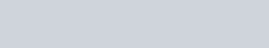Precipitation. Whenever the product of concentrations (raised to appropriate power) exceeds the solubility product, precipitation occurs.Common ion effects. Suppression of dissociation by adding an ion common with dissociation products. \text { e.g. } \mathrm{Ag}^{+} \text {or } \mathrm{C}_{2} \mathrm{O}_{4}^{2-} in the above example.Simultaneous solubility. While solving these problems, go as per general method i.e.(i) First apply condition of electroneutrality and (ii) Apply the equilibria conditions.
Thermodynamics Short Notes for Class 11, JEE & NEET
eSaral provides chemistry short notes for JEE and NEET to help students in revising topics quickly. These notes are completely based on latest syllabus and it includes all the tips and tricks that will help you in learning chemistry better and score well.The Notes will help you to understand the important topics and remember the key points for exam point of view.You can also access detailed Notes of chemistry here.Download or View Detailed Notes for Chemistry Class 11thDownload or View Detailed Notes for Chemistry Class 12thSolutions Short Notes for Class 12, IIT-JEE & NEET
eSaral provides chemistry short notes for JEE and NEET to help students in revising topics quickly. These notes are completely based on latest syllabus and it includes all the tips and tricks that will help you in learning chemistry better and score well.The Notes will help you to understand the important topics and remember the key points for exam point of view.You can also access Detailed Notes of chemistry here.Download or View Detailed Notes for Chemistry Class 11thDownload or View Detailed Notes for Chemistry Class 12thGaseous State Short Notes for Class 12, IIT-JEE & NEET
eSaral provides chemistry short notes for JEE and NEET to help students in revising topics quickly. These notes are completely based on latest syllabus and it includes all the tips and tricks that will help you in learning chemistry better and score well.The Notes will help you to understand the important topics and remember the key points for exam point of view.You can also access detailed Notes of chemistry here.Download or View Detailed Notes for Chemistry Class 11thDownload or View Detailed Notes for Chemistry Class 12thChemical Kinetics Short Notes for Class 12, IIT-JEE & NEET
eSaral provides chemistry short notes for JEE and NEET to help students in revising topics quickly. These notes are completely based on latest syllabus and it includes all the tips and tricks that will help you in learning chemistry better and score well.The Notes will help you to understand the important topics and remember the key points for exam point of view.You can also access detailed Notes of chemistry here.Download or View Detailed Notes for Chemistry Class 11thDownload or View Detailed Notes for Chemistry Class 12thCHEMISTRYCHEMICAL KINETICSTHEORYIt is a branch of physical chemistry deals with the “Rate of Chemical Reactions” including the effect of temperature, pressure, concentration, etc., on the rates, and the mechanism by which the reaction takes place.Rate of chemical Reaction is defined as the change in concentration of a reactant (or a product) in a particular time interval. Average rate of reaction, Instantaneous rate of reaction.Units of Reaction Rate are unit of concentration divided by the unit of time $\left(\mathrm{mol} \mathrm{L}^{-1} \mathrm{s}^{-1} \text { or } \mathrm{mol} \mathrm{L}^{-1} \mathrm{min}^{-1} \text { or so on }\right)$Factors Affecting Reaction Rates :(i) Concentration of reactants and(ii) Reaction temperatureBesides these, presence of catalyst and surface area (if a reactant or a catalyst is a solid) exposure to radiation also affect the reaction rates.Expressions or the rate :For a general reaction $: a A+b B \longrightarrow c C+d D$The rate of disappearance of A $=-\frac{\mathrm{d}[\mathrm{A}]}{\mathrm{dt}}$; Rate of disappearance of B $=-\frac{\mathrm{d}[\mathrm{B}]}{\mathrm{dt}}$ ;Rate of appearance of C = $\frac{\mathrm{d}[\mathrm{C}]}{\mathrm{dt}}$ & Rate of appearance of D = $\frac{\mathrm{d}[\mathrm{D}]}{\mathrm{dt}}$.The positive sign shows that concentrations of C and D increases with time and the negative sign indicating that concentrations of A and B decrease with time.Thus the rate of general reaction.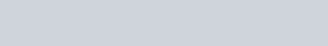Rate Equation and Rate constant :An expression which relates the rate of a reaction to the concentration of the reactants is called the Rate Equation or Rate Law. Rate $\propto[\mathrm{A}]^{\mathrm{a}} \cdot[\mathrm{B}]^{\mathrm{b}}$ or Rate = Rate $=\mathrm{k}[\mathrm{A}]^{\mathrm{a}}[\mathrm{B}]^{\mathrm{b}}$ . The constant of proportionality, k is known as the Rate Constant (specific reaction rate) and may be defined as the rate at unit concentrations of the reactants. k depends on the temperature and is independent of the initial concentrations of the reactants. At a fixed temperature, k is constant characteristic of the reaction. Larger value of k indicates fast reaction and small k indicates slow reactions.Molecularity :Molecularity of a reaction is defined as the numbers of particles (atoms, ions, groups or molecules) of reactants actually taking part in a single step chemical reaction.Molecularity of a reaction is :(i) Always a whole number (not zero) and never a fraction.(ii) The value of molecularity of a simple or one step reaction does not exceed 3.Order of Reaction :It is defined as the sum of the exponents (powers) of the molar concentrations of the reactants in the experimentally determined rate equations.If rate of reaction a $[\mathrm{A}]^{\mathrm{p}}[\mathrm{B}]^{\mathrm{q}}[\mathrm{C}]^{\mathrm{r}}$ or Rate of reaction $=\mathrm{k}[\mathrm{A}]^{\mathrm{p}}[\mathrm{B}]^{\mathrm{q}}[\mathrm{C}]^{\mathrm{r}}$order of reaction = p + q + r & the order w.r.t. A, B & C are p, q & r respectively.For a “Reaction of nth order”, the order of the reaction is n and the rate equation (or Rate law) is rate $\propto[\mathrm{A}]^{\mathrm{n}}=\mathrm{k}[\mathrm{A}]^{\mathrm{n}}$The order of a reaction is obtained from the experimentally determined rate (and not from the stoichiometric equation) and may be zero, an integer or a fraction and never exceeds 3. In a multi-step complex reaction, the order of the reaction depends on the slowest step.Zero order reaction :A reaction is said to be of zero order if the rate is independent of the concentration of the reactants.$A \longrightarrow$ products $;$ Rate $\alpha \mathrm{k}[\mathrm{A}]^{\circ}=\mathrm{k} \operatorname{mol} \mathrm{L}^{-1} \mathrm{s}^{-1}$Examples :(i) $\quad \mathrm{H}_{2}(\mathrm{g})+\mathrm{Cl}_{2}(\mathrm{g}) \stackrel{\mathrm{hv}}{\longrightarrow} 2 \mathrm{HCl}(\mathrm{g})$(ii) $\quad \mathrm{N}_{2} \mathrm{O}(\mathrm{g}) \frac{\text { hot Pt. }}{\text { Surface }} \mathrm{N}_{2}(\mathrm{g})+\frac{1}{2} \mathrm{O}_{2}(\mathrm{g})$(iii) $2 \mathrm{NH}_{3}$ (g) $\frac{\text { Mo or } \mathrm{W}}{\text { surface }} \mathrm{N}_{2}+3 \mathrm{H}_{2}$(iv) $\quad 2 \mathrm{HI}(\mathrm{g}) \frac{\mathrm{Au}}{\text { surface }} \mathrm{H}_{2}(\mathrm{g})+\mathrm{I}_{2}(\mathrm{g})$Characteristics of Zero Order Reaction :(1)Concentration of reactant decreases lineraly with time. $[\mathrm{A}]_{\mathrm{t}}=[\mathrm{A}]_{0}-\mathrm{kt}$(2) Units of k are, mol $l^{-1} \operatorname{time}^{-1}$(3) Time required for the completion of reaction t = $\frac{[\mathrm{A}]_{0}}{\mathrm{k}} \& \mathrm{t}_{1 / 2}=\frac{0.5[\mathrm{A}]_{0}}{\mathrm{k}}$First order reaction :a reaction is said to be of first order if its rate is proportinal to the concentration of one reactant only.Characteristics Of First Order Reaction :Examples :(i) Radioactive disintegration is a first order reaction.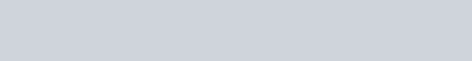(iii) Mineral acid catalyzed hydrolysis of esters.(iv) Decomposition of $H_{2} O_{2}$ in aqueous solution.Second Order Reaction :(i) When two molecules of the same reactant are involved or the concentrations of the both reactants are equal reactions $2 \mathrm{A} \longrightarrow$ products or $\mathrm{A}+\mathrm{B} \longrightarrow$ products.Characteristics of Second Order Reaction :(i) Unit of rate constant L $\mathrm{mol}^{-1}$ time $^{-1}$(ii) Numerical value of k will depend upon unit of concentration.(iii) $\left.\mathrm{t}_{1 / 2} \propto \mathrm{a}^{-1} \text { (In general } \mathrm{t}_{1 / 2} \alpha \mathrm{a}^{(1-\mathrm{n})} ; \mathrm{n}=\text { order of reactions }\right)$(iv) 2nd order reaction conforms to first order when one of the reactant in excess.Examples :Side or concurent reaction :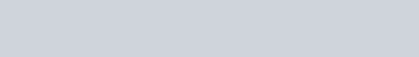Consecutive reaction :Threshold Energy and Activation Energy :For a reaction to take place the reacting molecules must colloid together, but only those collisions, in which colliding molecules possess certain minimum energy is called threshold energy $\left(\mathrm{E}_{\mathrm{T}}\right)$.Activation Energy $\left(\mathbf{E}_{\mathbf{a}}\right):$The extra energy needed for the reactant molecules to be able to react chemically is known as Activation energy.Influence of Temperature on reaction rates :Temperature coefficient :The temperature coefficient of a chemical reaction is defined as the ratio of the reaction rates at two temperatures differing by $10^{\circ} \mathrm{C}$. Its value usually lies between 2 & 3.Temperature coefficient $=\frac{\mathrm{k}_{\mathrm{t}+10}}{\mathrm{k}_{\mathrm{t}}}$Arrhenius Equation :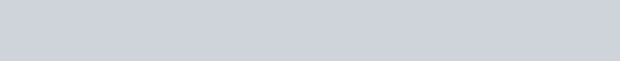Graphical representations are :Methods of Determination of order of reactions :A few methods commonly used are given below :1. Hit & Trial Method : It is method of using integrated rate equations, where the experimental values of a, x & t are put into these equations. One which gives a constant value of k for different sets of a, x & t correspond to the order of the reaction.2. Graphical Method :(i) A plot of log (a – x) versus ‘t’ gives a straight lines for the First order reaction.(ii) A plot of (a – x)– (n–1) versus ‘t’ gives a straight line any reaction of the order n (except n = 1)3.Half Life Method : The half life of different order of reactions is given by $\mathrm{a}_{\mathrm{n}}=\left(\frac{1}{2}\right)^{\mathrm{n}} \mathrm{a}_{0}$By experimental observation of the dependence of half life on initial concentration we can determine n, the order of reaction. N $=1+\frac{\log \mathrm{t}_{2}-\log \mathrm{t}_{1}}{\log \mathrm{a}_{1}-\log \mathrm{a}_{2}}$4. Initial rate method. Initial rate method is used to determine the order or reaction in cases where more than one reactant is used. It involves the determination of the order of different reactants separately. A series of experiments are performed in which concentration of one particular reactant is varied whereas conc. of other reactants are kept constant. In each experiment the initial rate is determined from the plot of conc. vs. time, e.g., if conc. of A is doubled, and initial rate of reaction is also doubled, order of reaction is l.Mechanism Of reactions :The path way which reactants are converted into the products is called the reaction mechanism. It should be clear that experimentally determined rate expression cannot be predicted from the stiochiometry of the reaction. For example for the reaction ;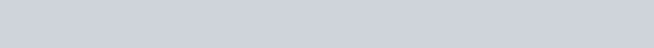The reason is that the reaction occurs by a series of elementary steps.The sequence of elementary processes leading to the overall stiochiometry is known as the “Mechanism of the reaction”. An in a sequence of reactions leading to the formation of products from reactants, the slowest step is the rate determining step.The mechanism proposed for the above reaction is a two step one.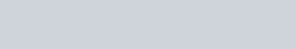The sum of the two gives the stiochiometry & the slow step decided the rate expression.
Electro Chemistry Short Notes for Class 12, IIT-JEE & NEET
eSaral provides chemistry short notes for JEE and NEET to help students in revising topics quickly. These notes are completely based on latest syllabus and it includes all the tips and tricks that will help you in learning chemistry better and score well.The Notes will help you to understand the important topics and remember the key points for exam point of view.You can also access detailed Notes of chemistry here.Download or View Detailed Notes for Chemistry Class 11thDownload or View Detailed Notes for Chemistry Class 12th Electrochemical cellsAn electrochemical cell consists of two electrodes (metallic conductors) in contact with an electrolyte (an ionic conductor).An electrode and its electrolyte comprise an Electrode Compartment. Electrochemical Cells can be classified as:(i) Electrolytic Cells in which a nonspontaneous reaction is driven by an external source of current.(ii) Galvanic Cells which produce electricity as a result of a spontaneous cell reactionNote : In a galvanic cell, cathode is positive with respect to anode.In a electrolytic cell, anode is made positive with respect to cathode.ElectrolysisThe decomposition of electrolyte solution by passage of electric current, resulting into deposition of metals or liberation of gases at electrodes is known as electrolysis.Electrolytic CellThis cell converts electrical energy into chemical energy. The entire assembly except that of the external battery is known as the electrolytic cellElectrodesThe metal strip at which positive current enters is called anode; anode is positively charged in electrolytic cell. On the other hand, the electrode at which current leaves is called cathode. Cathodes are negatively charged.Electrolysis of Molten sodium chloride$\mathrm{NaCl}(\mathrm{molten}) \longrightarrow \mathrm{Na}^{+}+\mathrm{Cl}^{-}$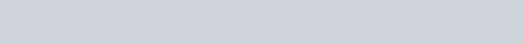There are two types of electrodes used in the electroytic cell, namely attackable and non – attackable. The attackable elecrodes participitate in the electrode reaction. They are made up of reactive metals like Zn, Cu, Ag etc. In such electrodes, atom of the metal gets oxidised into the corresponding cation, which is passed into the solution. Thus, such anodes get dissolved and their mass decreases. On the other hand, non-attackable electrodes do not participiate in the electrode reaction as they made up of unreactive elements like Pt, graphite etc. Such electrodes do not dissolve and their mass remain same.Faraday’s laws of electrolysis:(i) First law of electrolysis :Amount of substance deposited or liberated at an electrode is directly proportional to amount of charge passed (utilized) through the solution.$\mathrm{w} \propto \mathrm{Q}$W = weight liberated, Q = charge in coulombw = ZQZ = electrochemical equivalentwhen Q = 1 coulomb, then w = ZThus, weight deposited by 1 coulomb charge is called electrochemical equivalent.Let 1 ampere current is passed till ‘t’ seconds .Then, Q = It w = ZIt1 Faraday = 96500 coulomb = Charge of one mole electronsOne faraday is the charge required to liberate or deposit one gm equivalent of a substance at corresponding electrode.Let ‘E’ is equivalent weight then ‘E’ gm will be liberated by 96500 coulomb.$\therefore 1$ Coulomb will liberate $\frac{\mathrm{E}}{96500} \mathrm{gm} ;$ By definition, $Z=\frac{\mathrm{E}}{96500}$$\therefore W=\frac{I t E}{96500}When a gas is evolved at an electrode, then above formula changes as,\mathrm{V}=\frac{\mathrm{ItV}_{\mathrm{e}}}{96500}where V = volume of liberated gas, \mathrm{V}_{\mathrm{e}} = equivalent volume of gas.Equivalent volume may be defined as:The volume of gas liberated by 96500 coulomb at STP.(ii) Second law of electrolysis :When same amount of charge is passed through different electrolyte solutions connected in series then weight of substances deposited or dissolved at anode or cathode are in ratio of their equivalent weights. i.e.\mathrm{w}_{1} / \mathrm{w}_{2}=\mathrm{E}_{1} / \mathrm{E}_{2}QUALITATIVE ASPECTS OF ELECTROLYSISIn the electrolysis process we have discussed above, we have taken molten salt as electrolyte, which contains only one cation and anion. Now, if the electrolyte taken contains more than one cation and anion (for example, aqueous solution of the ionic electrolyte), then the cation and anion that will get discharged depends on the ability of cation to get reduced and the ability of anion to get oxidised.The ability of an ion to get oxidised or reduced depends upon the size, mass, positive charge, negative charge etc. Thus, it is not possible to predict qualitatively that which ion would be discharged first, a one factor might enhance the ability to discharge while the other factor may hamper it. This can only be predicted on the basis of quantitative value assigned based on the cumulative effect of all the factors reponsible for an ion’s ability to discharge. The value is referred as standard potential, which is determined by keeping the concentration of ion as 1 M, pressure of gas at 1 atm, and the measurement done at 25^{\circ} \mathrm{C}. For a cation, the standard reduction potential (SRP) values are compared. The cation having higher standard reduction potential value is discharged in preference to cation with lower SRP value provided the ions are at 1 M concentration. For an anion, the standard oxidation potential (SOP) values are compared and anion having higher SOP is preferentially discharged, if the concentration is 1 M for each of the ion. The SRP values at 25^{\circ} \mathrm{C}for some of the reduction half reactions are given in the table below.When solution of an electroyte contains more than one type of cations and anions at concentrations different than 1 M, the discharge of an ion does not depend solely on standard potentials but also depends on the concentration of ion in the solution. This value is refered as potential, called as reduction potential for cation and oxidation potential for anion. The relation between reduction potential and standard reduction potential is given by Nernst equation, as\mathrm{E}_{\mathrm{RP}}=\mathrm{E}_{\mathrm{RP}}^{\circ}-\frac{\mathrm{RT}}{\mathrm{nF}} \ln \frac{[\text { concentration of product }]}{[\text { concentration of reac tan } \mathrm{t}]}where E_{R P} = Reduction potential of cation and \mathrm{E}^{\mathrm{O}} \mathrm{RP}= Standard reduction potential of cation.Thus, it is possible that a cation (A+) with lower standard reduction potential getting discharged in preference to cation \left(\mathrm{B}^{+}\right) having higher standard reduction potential because their concentration might be such that the reduction potential of \mathrm{A}^{+} is higher than that of \mathrm{B}^{+}.When two metal ions in the solution have identical values of their reduction potentials, the simultaneous deposition of both the metals will occur in the form of an alloy.Galvanic Cell This cell converts chemical energy into electrical energy.Galvanic cell is made up of two half cells i.e., anodic and cathodic. The cell reaction is of redox kind. Oxidation takes place at anode and reduction at cathode. It is also known as voltaic cell. It may be represented as shown in Fig. Zinc rod immersed in \mathrm{ZnSO}_{4} behaves as anode and copper rod immersed in \mathrm{CuSO}_{4} behaves as cathode.Oxidation takes place at anode:\mathrm{Zn}^{3 / 4} \rightarrow \mathrm{Zn}^{2+}+2 \mathrm{e}^{-} (loss of electron : oxidation)Reduction takes place at cathode:\mathrm{Cu}^{2+}+2 \mathrm{e}^{-} \longrightarrow \mathrm{Cu}(\text { gain of electron } ; \text { reduction })Over all process:\mathrm{Zn}(\mathrm{s})+\mathrm{Cu}^{2+} \longrightarrow \mathrm{Cu}(\mathrm{s})+\mathrm{Zn}^{2+}In galvanic cell like Daniell cell; electrons flow from anode (zinc rod) to the cathode (copper rod) through external circuit; zinc dissolves as \mathrm{Zn}^{2+} ; \mathrm{Cu}^{2+}ion in the cathode cell picks up two electron and become deposited at cathode.Salt BridgeTwo electrolyte solutions in galvanic cells are seperated using salt bridge as represented in the Fig. salt bridge is a device to minimize or eliminate the liquid junction potential. Saturated solution of salt like KCI, \mathrm{KNO}_{3}, \mathrm{NH}_{4} \mathrm{Cl} and \mathrm{NH}_{4} \mathrm{NO}_{3} etc. in agar-agar gel is used in salt bridge. Salt bridge contains high concentration of ions viz. \mathrm{K}^{+} and \mathrm{NO}_{3}^{-}at the junction with electrolyte solution. Thus, salt bridge carries whole of the current across the boundary ; more over the \mathrm{K}^{+} and \mathrm{NO}_{3}^{-} ions have same speed. Hence, salt bridge with uniform and same mobility of cations and anions minimize the liquid junction potential & completes the electrical circuit & permits the ions to migrate.Representation of a cell (IUPAC conventions ): Let us illustrate the convention taking the example of Daniel cell.(i) Anodic half cell is written on left and cathodic half cell on right hand sid\mathrm{Zn}(\mathrm{s})\left|\mathrm{ZnSO}_{4}(\mathrm{sol}) \| \mathrm{CuSO}_{4}(\mathrm{sol})\right| \mathrm{Cu}(\mathrm{s})(ii) Two half cells are separated by double vertical lines: Double vertical lines indicate salt bridge or any type of porous partition.(iii) EMF (electromotive force) may be written on the right hand side of the cell.(iv) Single vertical lines indicate the phase separation between electrode and electrolyte solution.\mathrm{Zn}\left|\mathrm{Zn}^{2+} \| \mathrm{Cu}^{2+}\right| \mathrm{Cu}Concept of Electromotive force (EMF) of a CellElectron flows from anode to cathode in external circuit due to a pushing effect called or electromotic force (e.m.f.). E.m.f. is some times called as cell potential. Unit of e.m.f. of cell is volt.EMF of cell may be calculated as :E_{\operatorname{cell}} reduction potential of cathode – Reduction potential of anodeSimilarly, standard e.m.f. of the cell \left(\mathrm{E}^{\circ}\right) may be calculated as\mathrm{E}_{\text {cell }}^{\circ} = Standard reduction potential of cathode – Standard reduction potential of anodeSign Convention of EMFEMF of cell should be positive other wise it will not be feasible in the given direction .\begin{array}{ll}{\mathrm{Zn}\left|\mathrm{ZnSO}_{4} \| \mathrm{CuSO}_{4}\right| \mathrm{Cu}} & {\mathrm{E}=+1.10 \text { volt } \quad \text { (Feasible) }} \\ {\mathrm{Cu}\left|\mathrm{CuSO}_{4} \| \mathrm{ZnSO}_{4}\right| \mathrm{Zn}} & {\mathrm{E}=-1.10 \text { volt } \quad \text { (Not Feasible) }}\end{array}Nernst EquationWalter Nernst derived a relation between cell potential and concentration or Reaction quotient.\Delta \mathrm{G}=\Delta \mathrm{G}^{\circ}+\mathrm{R} \mathrm{T} \ln \mathrm{Q} \ldots .(1)where \Delta \mathrm{G} and \Delta \mathrm{G}^{\circ}are free energy and standard free energy change; ‘Q’ is reaction quotient.Let n, Faraday charge is taken out from a cell of e.m.f. (E) then electrical work done by the cell may be calculated as,Work done = Charge x Potential = nFEFrom thermodynamics we know that decrease in Gibbs free energy of a system is a measure of reversible or maximum obtainable work by the system if there is no work due to volume expans\therefore-\Delta \mathrm{G}=n \mathrm{FE} and -\Delta \mathrm{G}^{\circ}=n \mathrm{FE}^{\circ}Thus from Eq. (i), we get -n \mathrm{FE}=-n \mathrm{FE}^{\circ}+\mathrm{RT} \ln \mathrm{Q}At 25^{\circ} \mathrm{C}, above equation may be written as \mathbf{E}=\mathbf{E}^{\mathbf{0}}-\frac{0.0591}{\mathbf{n}} \log \mathbf{Q}Where ‘n’ represents number of moles of electrons involved in process.E, E° are e.m.f. and standard e.m.f. of the cell respectively.In general , for a redox cell reaction involving the transference of n electrons\mathrm{aA}+\mathrm{bB} \longrightarrow \mathrm{cC}+\mathrm{dD}, the EMF can be calculated as:\mathrm{E}_{\mathrm{Cell}}=\mathrm{E}_{\mathrm{Cell}}^{\circ}-\frac{0.0591}{\mathrm{n}} \log \frac{[\mathrm{C}]^{\mathrm{c}}[\mathrm{D}]^{\mathrm{d}}}{[\mathrm{A}]^{\mathrm{a}}[\mathrm{B}]^{\mathrm{b}}}Prediction and feasibility of spontaniety of a cell reaction.Work done by the cell = nFE;It is equivalent to decrease in free energy \Delta \mathrm{G}=-\mathrm{nFE}Under standard state \Delta \mathrm{G}^{0}=-\mathrm{nFE}^{0} ….. (i)(i) From thermodynamics we know, \DeltaG = negative for spontaneous process. Thus from eq.(i) it is clear that the EMF should be +ve for a cell process to be feasible or spontaneous.(ii) When \Delta \mathrm{G} = positive, E = negative and the cell process will be non spontaneous.Standard free energy change of a cell may be calculated by electrode potential data.Substituting the value of \mathrm{E}^{0} (i.e., standard reduction potentialof cathode- standard reduction potential of anode) in eq. (i) we may get \Delta \mathrm{G}^{0}Let us see whether the cell (Daniell) is feasible or not: i.e. whether Zinc will displace copper or not.\mathrm{Zn}|(\mathrm{s})| \mathrm{ZnSO}_{4}(\mathrm{sol}) \| \mathrm{CuSO}_{4}(\mathrm{sol}) | \mathrm{Cu}(\mathrm{s})$$\mathrm{E}_{\mathrm{Zn}^{2+} / \mathrm{Zn}}^{0}=-0.76 \mathrm{volt} ; \mathrm{E}_{\mathrm{Cu}^{2+} / \mathrm{Cu}}^{0}=+0.34 \mathrm{volt}$$\mathrm{E}_{\mathrm{cell}}^{0}=\mathrm{E}_{\mathrm{Cu}^{2+} / \mathrm{Cu}}^{0}-\mathrm{E}_{\mathrm{zn}^{2+} / \mathrm{Zn}}^{0}$$=0.34-(-0.76)=+1.10 \mathrm{volt}$Since $\mathrm{E}^{0}=+\mathrm{ve}$, hence the cell will be feasible and zinc will displace copper from its salt solution. In the other words zinc will reduce copper.8 Thermodynamic treatment of Nernst equationDetermination of equilibrium constant : We know, that$\mathrm{E}=\mathrm{E}^{0}-\frac{0.0591}{\mathrm{n}} \log \mathrm{Q}$ ..(i)At equilibrium, the cell potential is zero because cell reactions are balanced, i.e. E = 0$\therefore$ From Eq. (i), we have$0=\mathrm{E}^{0}-\frac{0.0591}{\mathrm{n}} \log \mathrm{K}_{\mathrm{eq}} \quad$ or $\quad \mathrm{K}_{\mathrm{eq}}=$ antilog $\left[\frac{n \mathrm{E}^{0}}{0.0591}\right]$Heat of Reaction inside the cell: Let n Faraday charge flows out of a cell of e.m.f. E, then$-\Delta \mathrm{G}=n \mathrm{FE}$ ……. (i)Gibbs Helmholtz equation (from thermodynamics ) may be given as,$\Delta \mathrm{G}=\Delta \mathrm{H}+\mathrm{T}\left[\frac{\partial \Delta \mathrm{G}}{\partial \mathrm{T}}\right]_{\mathrm{p}}$ ……….. (ii)From Eqs. (i) and (ii), we have$-\mathrm{nFE}=\Delta \mathrm{H}+\mathrm{T}\left[\frac{\partial(-\mathrm{nFE})}{\partial \mathrm{T}}\right]_{\mathrm{p}}=\Delta \mathrm{H}-\mathrm{nFT}\left[\frac{\partial \mathrm{E}}{\partial \mathrm{T}}\right]_{\mathrm{p}}$$\therefore \Delta \mathrm{H}=-\mathrm{nFE}+\mathrm{nFT}\left[\frac{\partial \mathrm{E}}{\partial \mathrm{T}}\right]_{\mathrm{p}}Entropy change inside the cell : We know that G = H – TS or DG = DH – TDS …(i)where \Delta \mathrm{G} = Free energy change ; DH = Enthalpy change and DS = entropy change.According to Gibbs Helmoholtz equation,\Delta \mathrm{G}=\Delta \mathrm{H}+\mathrm{T}\left[\frac{\partial \Delta \mathrm{G}}{\partial \mathrm{T}}\right]_{\mathrm{p}}$$\Delta \mathrm{G}=\Delta \mathrm{H}=\mathrm{T}\left[\frac{\partial \Delta \mathrm{G}}{\partial \mathrm{T}}\right]_{\mathrm{p}}$From Eqs. (i) and (ii), we have$-\mathrm{T} \Delta \mathrm{S}=\mathrm{T}\left[\frac{\partial \Delta \mathrm{G}}{\partial \mathrm{T}}\right]_{\mathrm{p}}$ or $\quad \Delta \mathrm{S}=-\left[\frac{\partial \Delta \mathrm{G}}{\partial \mathrm{T}}\right]_{\mathrm{p}}$$-\mathrm{T} \Delta \mathrm{S}=\mathrm{T}\left[\frac{\partial \Delta \mathrm{G}}{\partial \mathrm{T}}\right]_{\mathrm{p}} or \quad \Delta \mathrm{S}=-\left[\frac{\partial \Delta \mathrm{G}}{\partial \mathrm{T}}\right]_{\mathrm{p}}where \left[\frac{\partial \mathrm{E}}{\partial \mathrm{T}}\right]_{\mathrm{p}} is called temperature coefficient of cell e.m.f.8 Different Types of Half-Cells And Their Reduction Potential(1) Gas-Ion Half Cell:In such a half cell, an inert collector of electrons, platinum or graphite is in contact with gas and a solution containing a specified ion. One of the most important gas-ion half cell is the hydrogen-gas-hydrogen ion half cell. In this half cell, purified \mathrm{H}_{2} \mathrm{gas} at a constant pressure is passed over a platinum electrode which is in contact with an acid solution.\mathrm{H}^{+}(\mathrm{aq})+\mathrm{e}^{-} \rightleftharpoons 1 / 2 \mathrm{H}_{2}$$\mathrm{E}_{\mathrm{H}^{+} / \mathrm{H}_{2}}=\mathrm{E}^{0}_{\mathrm{H}^{+} / \mathrm{H}_{2}}-\frac{0.0591}{1} \log \frac{\left(\mathrm{pH}_{2}\right)^{1 / 2}}{\left[\mathrm{H}^{+}\right]}$(2) Metal-Metal Ion Half Cell:This type of cell consist of a metal M in contact with a solution containing $M^{n+}$ ions.$\mathrm{M}^{\mathrm{n}+}(\mathrm{aq})+\mathrm{ne}^{-} \rightleftharpoons \mathrm{M}(\mathrm{s})$$\mathrm{E}_{\mathrm{M}^{\mathrm{n}+} / \mathrm{M}}=\mathrm{E}_{\mathrm{M}^{\mathrm{n}+} / \mathrm{M}}^{0}-\frac{0.0591}{\mathrm{n}} \log \frac{1}{\left[\mathrm{M}^{\mathrm{n}+}\right]}(3) Metal-Insoluble Salt – Anion Half Cell:In this half cell, a metal coated with its insoluble salt is in contact with a solution containing the anion of the insoluble salt. eg. Silver-Silver Chloride Half Cell:This half cell is represented as CI’AgCl/Ag. The eq\mathrm{AgCl}(\mathrm{s})+\mathrm{e}^{-} \rightleftharpoons \mathrm{Ag}(\mathrm{s})+\mathrm{Cl}(\text { aq })potential of such cells depends upon the concentration of anions. Such cells can be used as Reference Electrode.(4) Oxidation-reduction Half Cell:This type of half cell is made by using an inert metal collector, usually platinum, immersed in a solution which contains two ions of the same element in different states of oxidation. eg. \mathrm{Fe}^{2+}-\mathrm{Fe}^{3+} half cell.\mathrm{Fe}^{3+}(\mathrm{aq})+\mathrm{e}^{-} \rightleftharpoons \mathrm{Fe}^{2+}(\mathrm{aq})$$\mathrm{E}_{\mathrm{Fe}^{3+} / \mathrm{Fe}^{2+}}=\mathrm{E}_{\mathrm{Fe}^{3+} / \mathrm{Fe}^{2+}}^{0}-\frac{0.0591}{1} \log \frac{\left[\mathrm{Fe}^{2+}\right]}{\left[\mathrm{Fe}^{3+}\right]}$8 Concentration cellThe cells in which electrical current is produced due to transport of a substance from higherto lower concentration. Concentration gradient may arise either in electrode material or in electrolyte. Thus there are two types of concentration cell .(i) Electrode concentration cell(ii) Electrolyte concentration cellElectrode Gas concentration cell :$\mathrm{Pt}, \mathrm{H}_{2}\left(\mathrm{P}_{1}\right)\left|\mathrm{H}^{+}(\mathrm{C})\right| \mathrm{H}_{2}\left(\mathrm{P}_{2}\right), \mathrm{Pt}$Here, hydrogen gas is bubbled at two different partial pressures at electrode dipped in the solution of same electrolyte.Anode process: $1 / 2 \mathrm{H}_{2}\left(\mathrm{p}_{1}\right) \rightarrow \mathrm{H}^{+}(\mathrm{c})+\mathrm{e}^{-}$Cathode process $\quad \frac{\mathrm{H}^{+}(\mathrm{c})+\mathrm{e}^{-} \rightarrow 1 / 2 \mathrm{H}_{2}\left(\mathrm{p}_{2}\right)}{1 / 2 \mathrm{H}_{2}\left(\mathrm{p}_{1}\right) \rightarrow 1 / 2 \mathrm{H}_{2}\left(\mathrm{p}_{2}\right)}$$\therefore \quad \mathrm{E}=-\frac{2.303 \mathrm{RT}}{\mathrm{F}} \log \left[\frac{\mathrm{p}_{2}}{\mathrm{p}_{1}}\right]^{1 / 2}$or $\quad \mathrm{E}=\left[\frac{2.303 \mathrm{RT}}{2 \mathrm{F}}\right] \log \left[\frac{\mathrm{p}_{2}}{\mathrm{p}_{1}}\right], \operatorname{At} 25^{0} \mathrm{C}, \mathrm{E}=\frac{0.059}{2 \mathrm{F}} \log \left[\frac{\mathrm{p}_{1}}{\mathrm{p}_{2}}\right]$For spontanity of such cell reaction, $\mathrm{p}_{1}>\mathrm{p}_{2}$Electrolyte concentration cells:$\mathrm{Zn}(\mathrm{s})\left|\mathrm{ZnSO}_{4}\left(\mathrm{C}_{1}\right) \| \mathrm{ZnSO}_{4}\left(\mathrm{C}_{2}\right)\right| \mathrm{Zn}(\mathrm{s})$In such cells, concentration gradient arise in electrolyte solutions. Cell process may be givewn a$\mathrm{zn}(\mathrm{s}) \rightarrow \mathrm{Zn}^{2+}\left(\mathrm{C}_{1}\right)+2 \mathrm{e} \quad$ (Anodic process)$\frac{\mathrm{Zn}^{2+}\left(\mathrm{C}_{2}\right)+2 \mathrm{e} \rightarrow \mathrm{Zn}(\mathrm{s})}{\mathrm{Zn}^{2+}\left(\mathrm{C}_{2}\right) \rightarrow \mathrm{Zn}^{2+}\left(\mathrm{C}_{1}\right)}$ (Over all process)$\therefore$ From Nernst equation, we have$\mathrm{E}=0-\frac{2.303 \mathrm{RT}}{2 \mathrm{F}} \log \left[\frac{\mathrm{C}_{1}}{\mathrm{C}_{2}}\right]$ OR $\mathrm{E}=\frac{2.303 \mathrm{RT}}{2 \mathrm{F}} \log \left[\frac{\mathrm{C}_{2}}{\mathrm{C}_{1}}\right]$For spontanity of such cell reaction, $\mathrm{C}_{2}>\mathrm{C}_{1}$8 CONDUCTANCE Introduction: Both metallic and electrolytic conductors obey Ohm’s lawi.e. V = IRwhere V = Potential difference in volt; I = Current in ampere ; R = resistance in OhmWe know, resistance is directly proportional to length of conductor and inversely proportional to cross sectional area of the conductor.$\operatorname{R\propto} \frac{l}{\mathrm{A}} \quad$ or $\mathrm{R}=\rho \frac{l}{\mathrm{A}}$ (= Specific resistance )Specific resistance is the resistance of a conductor having lengths of 1 cm and corss sectional area of 1cm2.Unit of R is ohm and unit of specific resistance is ohm cmReciprocal of resistance is called as conductance and reciprocal of specific resistance is called as specific conductance.$\frac{1}{\mathrm{R}}=\frac{1}{\rho} \frac{\mathrm{A}}{l}$ or $\mathrm{C}=\mathrm{K} \frac{\mathrm{A}}{l}$where C = conductance ohm $^{-1}$; K = specific conductance ohm $^{-1} \mathrm{cm}^{-1}$Mho and siemens are other units of conductance.$\mathrm{K}=\frac{l}{\mathrm{A}} \mathrm{C}$Specific conductance= Cell constant x ConductanceSpecific conductance is conductance of 1 $\mathbf{C} \mathbf{M}^{3}$ of an electrolyte solution. In case of electrolytic solution, the specific conductance is defined as the conductance of a solsution of definite concentration enclosed in a cell having two electrodes sof unit area separated by 1 cm apart.1. Equivalent Conductance Equivalent conductance is the conductance of an electrolyte solution containing 1 gm equivalent of electrolyte. It is densoted by $\wedge$ .$\wedge$ = K x V$\left(\wedge=\operatorname{ohm}^{-1} \mathrm{cm}^{-1} \mathrm{x} \mathrm{cm}^{3}=\mathrm{ohm}^{-1} \mathrm{cm}^{2}\right)$Usually concentration of electrolyte solution is expressed as C gm equivalent per litre.Thus, $\mathrm{V}=\frac{1000}{\mathrm{C}}$2. Molar ConductanceMolar conductance may be defined as conductance of an electrolyte solution having 1 gm mole electrolyte in a litre. It is denoted by $\wedge_{\mathrm{m}}$ .$\wedge_{\mathrm{m}}=\mathrm{K} \times \mathrm{V}$Usually concentration of electrolyte solution is expressed as ‘M’ gm mole elecrtrolyte per litre.The $\dot{\mathrm{U}}_{\mathrm{m}}$ vs $\sqrt{\mathrm{C}}$ plot of strong electrolyte being linear it can be extrapolated to zero concentration. Thus, $\dot{\mathrm{U}}_{\mathrm{m}}$ values of the solution of the test electrolyte are determined at various concentrations the concentrations should be as low as good.$\dot{\mathrm{U}}_{\mathrm{m}}$ values are then plotted against $\sqrt{\mathrm{C}}$ when a straight line is obtained. This is the extrapolated to zero concentration. The point where the straight line intersects $\dot{\mathrm{U}}_{\mathrm{m}}$ axis is of the strong electrolyte.However, the plot in the case weak electrolyte being non linear, shooting up suddenly at some low concentration and assuming the shape of a straight line parallel to Ùm axis. Hence extrapolation in this case is not possible. Thus, $\dot{\mathrm{U}}_{0}$of a weak electrolyte cannot be determined experimentally. It can, however, be done with the help of Kohlrausch’s law to be discussed later.8 Kohlrausch’s Law of Independent Migration of IonsKohlrausch determined $\dot{\mathrm{U}}_{0}$ values of pairs of some strong electrolytes containing same cation say KF and KCl, NaF and NaCl etc., and found that the difference in $\dot{\mathrm{U}}_{0}$ values in each case remains the same:$\wedge_{\mathrm{m}}^{0}(\mathrm{KCl})-\wedge_{\mathrm{m}}^{0}(\mathrm{KF})=\wedge_{\mathrm{m}}^{0}(\mathrm{NaCl})-\wedge_{\mathrm{m}}^{0}(\mathrm{NaF})$He also detemined $\dot{\mathrm{U}}_{0}$ values of pairs of strong electrolytes containing same anion say KF and NaF, KCl and NaCl etc.and found that the difference in Ù0 values in each case remains the same.$\wedge_{\mathrm{m}}^{0}(\mathrm{KF})-\wedge_{\mathrm{m}}^{0}(\mathrm{NaF})=\wedge_{\mathrm{m}}^{0}(\mathrm{KCl})-\wedge_{\mathrm{m}}^{0}(\mathrm{NaCl})$At infinite dilution when dissociation is complete, every ion makes some definite contribution towards molar conductance of the electrolyte irrespective of the nature of the other ion which with it is associated and that the molar conductance at infinite dilution for any electrolyte is given by the sum of the contribution of the two ions. Thus,$\wedge_{\mathrm{m}}^{0}=\lambda_{+}^{0}+\lambda_{-}^{0}$Where $\lambda_{+}^{0}$ is the contribution of the cation and $\lambda_{-}^{0}$ is the contribution of the anion towards the molar conductance at infinite dilution. These contributions are called molar ionic conductances at infinite dilution. Thus, $\lambda_{+}^{0}$ is the molar ionic conductance of cation and$\lambda_{-}^{0}$ is the molar ionic conductnace of anion, at infinite dilution. The above equation is, however, correct only for binary electrolyte like NaCl, $\mathrm{MgSO}_{4}$ etc.8 Application of Kohlrausch’s law :(1) Determination of $\wedge_{\mathrm{m}}^{0}$ of a weak electrolyte:In order to calculate $\wedge_{\mathrm{m}}^{0}$ of a weak electrolyte say CH3COOH, we determine experimentally $\wedge_{\mathrm{m}}^{0}$ values ofthe following three strong electrolytes:(a) A strong electrolyte containing same cation as in the test electrolyte, say HCl(b) A strong electrolyte containing same anion as in the test electrolyte, say $\mathrm{CH}_{3} \mathrm{COONa}$(c) A strong electrolyte containing same anion of (a) and cation of (b) i.e. NaCl.$\wedge_{\mathrm{m}}^{0}$ of $\mathrm{CH}_{3} \mathrm{COOH}$ is then given as:$\wedge_{\mathrm{m}}^{0}\left(\mathrm{CH}_{3} \mathrm{COOH}\right)=\wedge_{\mathrm{m}}^{0}(\mathrm{HCl})+\wedge_{\mathrm{m}}^{0}\left(\mathrm{CH}_{3} \mathrm{COONa}\right)-\wedge_{\mathrm{m}}^{0}(\mathrm{NaCl})$Proof:$\wedge_{\mathrm{m}}^{0}(\mathrm{HCl})+\wedge_{\left(\mathrm{CH}_{3} \mathrm{COONa}\right)}^{0}-\wedge_{(\mathrm{NaCl})}^{0}=\lambda_{\left(\mathrm{H}^{+}\right)}^{0}+\lambda_{\left(\mathrm{CH}_{3} \mathrm{COO}^{0}\right)}^{0}=\wedge_{0\left(\mathrm{CH}_{3} \mathrm{COOH}\right)}$(2) Determination of degree of dissociation (a) :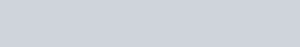(3) Determination of solubility of sparingly soluble saltThe specific conductivity of a saturated solution of the test electrolyte (sparingly soluble) made in conductivity water is determined by the method as described above. From this the specific conductivity of conductivity water is deducted. The molar conductance of the saturated solution is taken to be equal to $\wedge_{\mathrm{m}}^{0}$ as the saturated solution of a sparingly soluble salt is extremely dilute.$\wedge_{\mathrm{m}}^{0}=\frac{1000 \mathrm{\kappa}}{\mathrm{C}}$where C is the molarity of solution and hence the solubility.